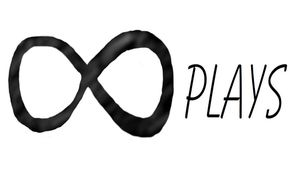By Bob

Making a parts box is fairly simple, but adding a label that automatically relocates when the box is resized is a bit tricky, here is a code example that allows you to resize the box, round the corners and add custom text.

``````/*[Parts box]*/
box_height = 20;//[20:.01:50]
box_size_x = 40;//[20:.01:200]
box_size_y = 40;//[20:.01:200]
wall_thickness = .5;//[.1:.01:1]
rounding = 40; //[4:1:40]

/*[Label]*/
InputText = "Label";
Font_Style = "";
TextSize= 4; //[4:0.01:6.5]
Text_Extrude = .50; //[0.10:0.01:.75]
TextSpacing = 1; //[1:0.01:1.5]

module box(){
translate([0,0,box_height/2])
difference(){
cube([box_size_x,box_size_y,box_height],center=true);
cube([box_size_x-wall_thickness,box_size_y-wall_thickness,box_height+2],center=true);
}
translate([0,0,wall_thickness/2])
cube([box_size_x,box_size_y,1],center=true);
rotate([90,0,90])
translate([box_size_y+2.5,box_height-.87,box_size_x/400])
translate([-box_size_y-box_size_y/2,0,-box_size_x/2])
scale([5,1,1])
cylinder(box_size_x-.1,1,1,\$fn=3,center=false);
}

module label(){
color("grey")
rotate(a = [-173,180,0])
linear_extrude(height = Text_Extrude)
text(InputText,font=Font_Style,TextSize,halign = "center",valign = "center",spacing = TextSpacing, \$fn=100);
}

minkowski(){
box();
sphere(wall_thickness,\$fn=rounding);
}
translate([0,0,box_height-.1])
translate([0,(-box_size_y/2+5)+(wall_thickness-1.3),0])
label();``````

If you aren't familiar with how to add text in OpenSCAD you goto Help>Font List:

Then select the font you want from the list and copy it to the clipboard:

Then paste it into the Font Style box in the customizer and remove the quotation marks:

Then the font style should change, I made another post on making a text module in post #11:

As with all of my projects I will be making changes and improvements...

## Related ArticlesFollow